# irfplot

Plot impulse response function (IRF) of state-space model

## Syntax

``irfplot(Mdl)``
``irfplot(Mdl,Name,Value)``
``irfplot(___,'Params',estParams)``
``irfplot(___,'Params',estParams,'EstParamCov',EstParamCov)``
``irfplot(ax,___)``
``h = irfplot(ax,___)``

## Description

`irfplot` plots the IRFs of the state and measurement variables in a state-space model. To return the IRFs as numeric arrays instead, use `irf`. Other state-space model tools to characterize the dynamics of a specified system include:

• The forecast error variance decomposition (FEVD), computed by `fevd`, provides information about the relative importance of each state disturbance in affecting the forecast error variance of all measurement variables in the system.

• Model-implied temporal correlations, computed by `corr` for a standard state-space model, measure the association between present and past state or measurement variables, as prescribed by the form of the model.

### Fully Specified State-Space Model

example

````irfplot(Mdl)` plots the IRF, or dynamic response, of each state and measurement variable of the fully specified state-space model `Mdl`, such as an estimated model. `irfplot` plots a figure containing the IRFs of the measurement variables yt, and plots a separate figure containing the IRFs of the state variables xt. Each figure contains a subplot for each variable and state disturbance combination; subplot (i,j) is the IRF of variable j resulting from a unit shock applied to a state disturbance i ui,t. Subplot titles identify the shocked variable and IRF variable.```

example

``irfplot(Mdl,Name,Value)` uses additional options specified by one or more name-value pair arguments. For example, `'PlotU',1:2,'PlotX',[]` plots only the measurement variable IRFs resulting from shocks applied to the first and second state-disturbance variables (the state variable IRF plot is suppressed).`

### Partially Specified State-Space Model and Confidence Interval Estimation

example

``irfplot(___,'Params',estParams)` plots the IRFs of the partially specified state-space model `Mdl` using any of the input argument combinations in the previous syntaxes. `estParams` specifies estimates of all unknown parameters in the model.`

example

``irfplot(___,'Params',estParams,'EstParamCov',EstParamCov)` also plots pointwise lower and upper 95% Monte Carlo confidence bounds in each plot. `EstParamCov` specifies the estimated covariance matrix of the parameter estimates, as returned by the `estimate` function, and is required for confidence interval estimation.`

### Customize Figures

example

``irfplot(ax,___)` plots on the axes objects specified by `ax` instead of new figures. The option `ax` can precede any of the input argument combinations in the previous syntaxes.`

example

``h = irfplot(ax,___)` also returns an array of plot handles `h`. Use `h` to modify properties of the plots after you create them.`

## Examples

collapse all

Explicitly create the state-space model

`$\begin{array}{l}{x}_{t}=0.5{x}_{t-1}+0.2{u}_{t}\\ {y}_{t}=2{x}_{t}+0.01{\epsilon }_{t}.\end{array}$`

```A = 0.5; B = 0.2; C = 2; D = 0.01; Mdl = ssm(A,B,C,D)```
```Mdl = State-space model type: ssm State vector length: 1 Observation vector length: 1 State disturbance vector length: 1 Observation innovation vector length: 1 Sample size supported by model: Unlimited State variables: x1, x2,... State disturbances: u1, u2,... Observation series: y1, y2,... Observation innovations: e1, e2,... State equation: x1(t) = (0.50)x1(t-1) + (0.20)u1(t) Observation equation: y1(t) = (2)x1(t) + (0.01)e1(t) Initial state distribution: Initial state means x1 0 Initial state covariance matrix x1 x1 0.05 State types x1 Stationary ```

`Mdl` is an `ssm` model object. Because all parameters have known values, the object is fully specified.

Plot the IRFs of the measurement and state variables.

`irfplot(Mdl);`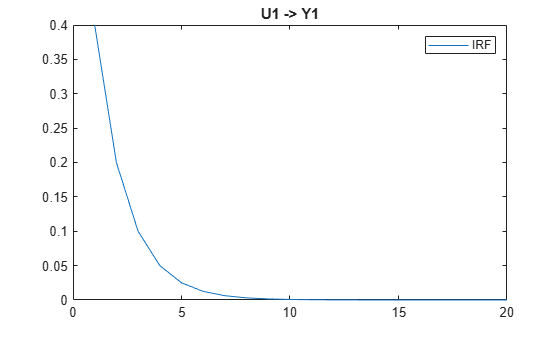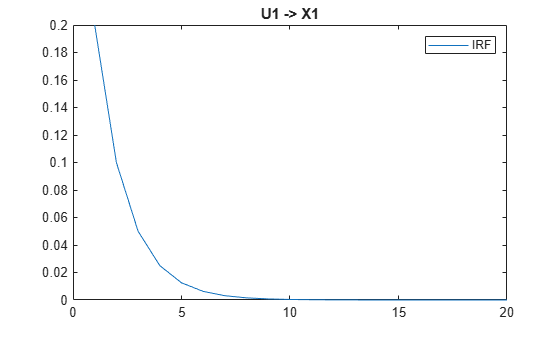The plot entitled U1 -> Y1 is the IRF of ${\mathit{y}}_{\mathit{t}}$, and the plot entitled U1 -> X1 is the IRF of ${\mathit{x}}_{\mathit{t}}$. Both IRFs indicate that the effects of the shock on the system diminish after about 8 periods.

Plot the 10-period IRFs of only the measurement variables in a system.

Explicitly create the multivariate diffuse state-space model

`$\begin{array}{l}{x}_{1,t}={x}_{1,t-1}+0.2{u}_{1,t}\\ {x}_{2,t}={x}_{1,t-1}+0.3{x}_{2,t-1}+{u}_{2,t}\\ {y}_{1,t}={x}_{1,t}+{\epsilon }_{1,t}\\ {y}_{2,t}={x}_{1,t}+{x}_{2,t}+{\epsilon }_{2,t}.\end{array}$`

```A = [1 0; 1 0.3]; B = [0.2 0; 0 1]; C = [1 0; 1 1]; D = eye(2); Mdl = dssm(A,B,C,D)```
```Mdl = State-space model type: dssm State vector length: 2 Observation vector length: 2 State disturbance vector length: 2 Observation innovation vector length: 2 Sample size supported by model: Unlimited State variables: x1, x2,... State disturbances: u1, u2,... Observation series: y1, y2,... Observation innovations: e1, e2,... State equations: x1(t) = x1(t-1) + (0.20)u1(t) x2(t) = x1(t-1) + (0.30)x2(t-1) + u2(t) Observation equations: y1(t) = x1(t) + e1(t) y2(t) = x1(t) + x2(t) + e2(t) Initial state distribution: Initial state means x1 x2 0 0 Initial state covariance matrix x1 x2 x1 Inf 0 x2 0 Inf State types x1 x2 Diffuse Diffuse ```

`Mdl` is a fully specified `ssm` model object.

Plot the two 10-period IRFs of ${\mathit{y}}_{2,\mathit{t}}$, and suppress the state variable IRFs.

`irfplot(Mdl,'NumPeriods',10,'PlotY',2,'PlotX',[]);`The top subplot is the IRF of ${\mathit{y}}_{2,\mathit{t}}$ resulting from a shock to ${\mathit{u}}_{1,\mathit{t}}$, which is persistent because the shock filters through the random walk state ${\mathit{x}}_{1,\mathit{t}}$.

The bottom subplot is the IRF of ${\mathit{y}}_{2,\mathit{t}}$ resulting from a shock to ${\mathit{u}}_{2,\mathit{t}}$, which is transient and eventually diminishes as time elapses because the state ${\mathit{x}}_{2,\mathit{t}}$ exhibits autoregressive behavior.

Simulate data from a known model, fit the data to a state-space model, and then the plot cumulative IRFs of the estimated model to specified axes.

Assume that the data generating process (DGP) is the AR(1) model

`${x}_{t}=1+0.75{x}_{t-2}+{u}_{t},$`

where ${\mathit{u}}_{\mathit{t}}$ is a series of independent and identically distributed Gaussian variables with mean 0 and variance 1.

Simulate 500 observations from the model.

```rng(1); % For reproducibility DGP = arima('Constant',1,'AR',{0 0.75},'Variance',1); y = simulate(DGP,500);```

Explicitly create a state-space model template for estimation that represents the model

`$\begin{array}{l}{x}_{t}=c+\varphi {x}_{t-2}+\eta {u}_{t}\\ {y}_{t}={x}_{t}.\end{array}$`

```A = [0 NaN NaN; 0 1 0; 1 0 0]; B = [NaN; 0; 0]; C = [1 0 0]; D = 0; Mdl = ssm(A,B,C,D,'StateType',[0 1 0]);```

Fit the model template to the data. Specify a set of positive, random standard Gaussian starting values for the three model parameters.

`EstMdl = estimate(Mdl,y,abs(randn(3,1)));`
```Method: Maximum likelihood (fminunc) Sample size: 500 Logarithmic likelihood: -892.214 Akaike info criterion: 1790.43 Bayesian info criterion: 1803.07 | Coeff Std Err t Stat Prob -------------------------------------------------- c(1) | 0.41320 0.12199 3.38730 0.00071 c(2) | 0.67319 0.02749 24.48749 0 c(3) | 1.11450 0.03623 30.76557 0 | | Final State Std Dev t Stat Prob x(1) | 3.69929 0 Inf 0 x(2) | 1 0 Inf 0 x(3) | 1.43378 0 Inf 0 ```

`EstMdl` is a fully specified `dssm` model object.

Plot the cumulative IRFs of the first and third state variables, and the measurement variable in `EstMdl`. Return the plot in the same figure, on three separate subplots.

```ax = gobjects(3,1); for j = 1:numel(ax) ax(j) = subplot(3,1,j); end irfplot(ax,EstMdl,'Cumulative',true,'PlotX',[1 3]);```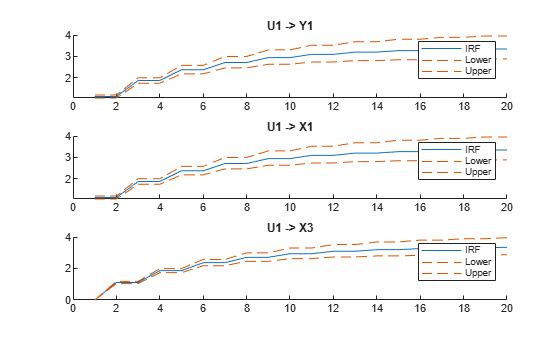Because ${\mathit{y}}_{\mathit{t}}={\mathit{x}}_{\mathit{t}}$, the top two IRFs in the figure are equivalent. Because ${\mathit{x}}_{1,\mathit{t}-1}={\mathit{x}}_{3,\mathit{t}}$, the IRF in the subplot at the bottom of the figure is shifted to the left, relative to the other two plots.

Simulate data from a time-varying state-space model, fit a model to the data, then plot the time-varying IRF of the estimated model.

Consider the DGP represented by this system

`$\begin{array}{l}{x}_{t}=\left\{\begin{array}{ll}0.75{x}_{t-1}+{u}_{t};& t<11\\ -0.1{x}_{t-1}+3{u}_{t};& t\ge 11\end{array}\\ {y}_{t}=1.5{x}_{t}+2{\epsilon }_{t}.\end{array}$`

The function `timeVariantAR1ParamMap.m`, stored in `mlr/examples/econ/main`, specifies the model structure. `mlr` is the value of `matlabroot`.

`type timeVariantAR1ParamMap.m`
```% Copyright 2020 The MathWorks, Inc. function [A,B,C,D] = timeVariantAR1ParamMap(params) % Time-varying state-space model parameter mapping function example. This % function maps the vector params to the state-space matrices (A, B, C, and % D). From periods 1 through 10, the state model is an AR(1)model, and from % periods 11 through 20, the state model is possibly a different AR(1) % model. The measurement equation is the same throughout the time span. A1 = {params(1)}; A2 = {params(2)}; varu1 = exp(params(3)); % Positive variance constraints varu2 = exp(params(4)); B1 = {sqrt(varu1)}; B2 = {sqrt(varu2)}; C = params(5); vare1 = exp(params(6)); D = sqrt(vare1); A = [repmat(A1,10,1); repmat(A2,10,1)]; B = [repmat(B1,10,1); repmat(B2,10,1)]; end ```

Implicitly create a partially specified state-space model representing the DGP. For this example, fix the measurement-sensitivity coefficient $\mathit{C}$ to `1.5`.

```C = 1.5; fixCParamMap = @(x)timeVariantAR1ParamMap([x(1:4), C, x(5)]); DGP = ssm(fixCParamMap);```

Simulate 20 observations from the DGP. Because `DGP` is partially specified, pass the true parameter values to `simulate` by using the `'Params'` name-value pair argument.

```rng(10) % For reproducibility A1 = 0.75; A2 = -0.1; B1 = 1; B2 = 3; D = 2; trueParams = [A1 A2 2*log(B1) 2*log(B2) 2*log(D)]; % Transform variances for parameter map y = simulate(DGP,20,'Params',trueParams);```

`y` is a 20-by-1 vector of simulated measurements ${\mathit{y}}_{\mathit{t}}$ from the DGP.

Because `DGP` is a partially specified, implicit model object, its parameters are unknown. Therefore, it can serve as a model template for estimation.

Fit the model to the simulated data. Specify random standard Gaussian draws for the initial parameter values. Return the parameter estimates.

`[~,estParams] = estimate(DGP,y,randn(1,5),'Display','off')`
```estParams = 1×5 0.6164 -0.1665 0.0135 1.6803 -1.5855 ```

`estParams` is a 1-by-5 vector of parameter estimates. The output argument list of the parameter mapping function determines the order of the estimates: `A{1}`, `A{2}`, `B{1}`, `B{2}`, and `D`.

Plot the IRF of the measurement and state variables by supplying `DGP` (not the estimated model) and the estimated parameters by using the `'Params'` name-value pair argument.

```h = irfplot(DGP,'Params',estParams); xline(h(1,1).Parent,10.5,'--')```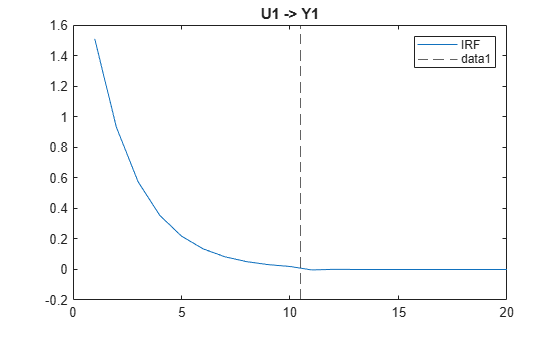`xline(h(1,2).Parent,10.5,'--')`The figures show time-varying IRFs of the measurement and state variables. The first 10 periods correspond to the IRF of the first state equation. During period 11, what remains of the shock transfers to the second state equation, and filters through that system until it diminishes.

Plot the measurement variuable IRF and the 95% confidence intervals on the true IRFs.

Assume that the data generating process (DGP) is the AR(1) model

`${x}_{t}=1+0.75{x}_{t-2}+{u}_{t},$`

where ${\mathit{u}}_{\mathit{t}}$ is a series of independent and identically distributed Gaussian variables with mean 0 and variance 1.

Simulate 500 observations from the model.

```rng(1); % For reproducibility DGP = arima('Constant',1,'AR',{0 0.75},'Variance',1); y = simulate(DGP,500);```

Explicitly create a diffuse state-space model template for estimation that represents the model. Fit the model to the data, and return parameter estimates and their corresponding estimated covariance matrix.

```A = [0 NaN NaN; 0 1 0; 1 0 0]; B = [NaN; 0; 0]; C = [1 0 0]; D = 0; Mdl = dssm(A,B,C,D,'StateType',[0 1 0]); [~,estParams,EstParamCov] = estimate(Mdl,y,abs(randn(3,1)));```
```Method: Maximum likelihood (fminunc) Effective Sample size: 500 Logarithmic likelihood: -892.214 Akaike info criterion: 1790.43 Bayesian info criterion: 1803.07 | Coeff Std Err t Stat Prob -------------------------------------------------- c(1) | 0.41320 0.12199 3.38730 0.00071 c(2) | 0.67319 0.02749 24.48749 0 c(3) | 1.11450 0.03623 30.76557 0 | | Final State Std Dev t Stat Prob x(1) | 3.69929 0 Inf 0 x(2) | 1 0 Inf 0 x(3) | 1.43378 0 Inf 0 ```

`Mdl` is a fully specified, estimated `dssm` model object.

Plot the IRF, with its 95% confidence intervals, of the measurement variable.

```irfplot(Mdl,'Params',estParams,'EstParamCov',EstParamCov,... 'PlotX',[]);```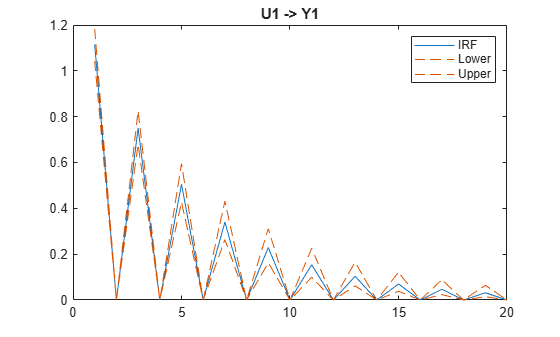The blue line represents the estimated IRF of ${\mathit{y}}_{\mathit{t}}$. The dashed red lines represent the upper and lower, pointwise 95% confidence bounds on the true IRF. The model has only one lag term (lag 2), as the shock filters through the system, it impacts the first state variable during odd periods only.

## Input Arguments

collapse all

State-space model, specified as an `ssm` model object returned by `ssm` or its `estimate` function, or a `dssm` model object returned by `dssm` or its `estimate` function.

If `Mdl` is partially specified (that is, it contains unknown parameters), specify estimates of the unknown parameters by using the `'Params'` name-value argument. Otherwise, `irfplot` issues an error.

`irfplot` issues an error when `Mdl` is a dimension-varying model, which is a time-varying model containing at least one variable that changes dimension during the sampling period (for example, a state variable drops out of the model).

Tip

If `Mdl` is fully specified, you cannot estimate confidence bounds. To estimate confidence bounds:

1. Create a partially specified state-space model template for estimation `Mdl`.

2. Estimate the model by using the `estimate` function and data. Return the estimated parameters `estParams` and estimated parameter covariance matrix `EstParamCov`.

3. Pass the model template for estimation `Mdl` to `irfplot`, and specify the parameter estimates and covariance matrix by using the `'Params'` and `'EstParamCov'` name-value arguments.

4. For the `irfplot` function, return the appropriate output arguments for lower and upper confidence bounds.

Axes on which to plot the IRFs, specified as a `pU*(pY + pX)`-by-`1` vector of `Axes` objects, where `pU`, `pY`, and `pX` are the lengths of the values of the `'PlotU'`, `'PlotY'`, and `'PlotX'` name-value pair arguments, respectively.

`irfplot` plots IRFs to the axes in `ax` in this order.

1. IRFs of all measurement variables `PlotY(:)` resulting from a shock to the first state disturbance `PlotU(1)`.

2. IRFs of all measurement variables `PlotY(:)` resulting from a shock to the second state disturbance `PlotU(2)`.

3. Continue the procedure similarly until `irfplot` plots the IRF associated with the last state disturbance `PlotU(end)`.

4. Repeat steps 1 through 3, but replace the measurement variables with the state variables `PlotX`.

By default, `irfplot` plots the measurement-variable IRFs to the axes of subplots in a new figure, and plots the state-variable IRFs to the axes of subplots in another new figure.

### Name-Value Arguments

Specify optional pairs of arguments as `Name1=Value1,...,NameN=ValueN`, where `Name` is the argument name and `Value` is the corresponding value. Name-value arguments must appear after other arguments, but the order of the pairs does not matter.

Before R2021a, use commas to separate each name and value, and enclose `Name` in quotes.

Example: `'PlotU',1:2,'PlotX',[]` plots only the measurement-variable IRFs resulting from shocks applied to the first and second state-disturbance variables (the state-variable IRF plot is suppressed).

IRF Options

collapse all

Number of periods for which `irfplot` computes the IRF, specified as a positive integer. Periods in the IRF start at time 1 and end at time `NumPeriods`.

Example: `'NumPeriods',10` specifies the inclusion of 10 consecutive time points in the IRF starting at time 1, during which `irfplot` applies the shock, and ending at time 10.

Data Types: `double`

Estimates of the unknown parameters in the partially specified state-space model `Mdl`, specified as a numeric vector.

If `Mdl` is partially specified (contains unknown parameters specified by `NaN`s), you must specify `Params`. The `estimate` function returns parameter estimates of `Mdl` in the appropriate form. However, you can supply custom estimates by arranging the elements of `Params` as follows:

• If `Mdl` is an explicitly created model (`Mdl.ParamMap` is empty `[]`), arrange the elements of `Params` to correspond to hits of a column-wise search of `NaN`s in the state-space model coefficient matrices, initial state mean vector, and covariance matrix.

• If `Mdl` is time invariant, the order is `A`, `B`, `C`, `D`, `Mean0`, and `Cov0`.

• If `Mdl` is time varying, the order is `A{1}` through `A{end}`, `B{1}` through `B{end}`, `C{1}` through `C{end}`, `D{1}` through `D{end}`, `Mean0`, and `Cov0`.

• If `Mdl` is an implicitly created model (`Mdl.ParamMap` is a function handle), the first input argument of the parameter-to-matrix mapping function determines the order of the elements of `Params`.

If `Mdl` is fully specified, `irfplot` ignores `Params`.

Example: Consider the state-space model `Mdl` with `A = B = [NaN 0; 0 NaN]` , `C = [1; 1]`, `D = 0`, and initial state means of 0 with covariance `eye(2)`. `Mdl` is partially specified and explicitly created. Because the model parameters contain a total of four `NaN`s, `Params` must be a 4-by-1 vector, where `Params(1)` is the estimate of `A(1,1)`, `Params(2)` is the estimate of `A(2,2)`, `Params(3)` is the estimate of `B(1,1)`, and `Params(4)` is the estimate of `B(2,2)`.

Data Types: `double`

State-disturbance variables ut to shock for the IRF plots, specified as the comma-separated pair consisting of `'PlotU'` and a vector of positive integers. Elements are the indices of the state-disturbance variables u1,t, u2,t, …, uk,t.

By default, `irfplot` shocks all state-disturbance variables.

Example: `'PlotU',[1 3]` shocks u1,1 and u3,1, and `irfplot` plots the resulting IRFs.

Data Types: `double`

Measurement-variable IRFs to plot, specified as the comma-separated pair consisting of `'PlotY'` and a vector of positive integers. Elements are the indices of the measurement variables y1,t, y2,t, …, yn,t.

If `PlotY` is empty `[]`, `irfplot` does not plot any measurement-variable IRFs.

By default, `irfplot` plots all measurement-variable IRFs.

Example: `'PlotY',1` plots the IRF of y1,t.

Data Types: `double`

State-variable IRFs to plot, specified as the comma-separated pair consisting of `'PlotX'` and a vector of positive integers. Elements are the indices of the state variables x1,t, x2,t, …, xm,t.

If `PlotX` is empty `[]`, `irfplot` does not plot any state-variable IRFs.

By default, `irfplot` plots all state-variable IRFs.

Example: `'PlotX',[]` does not plot any state-variable IRFs.

Data Types: `double`

Flag for computing the cumulative IRF, specified as a value in this table.

ValueDescription
`true``irfplot` computes the cumulative IRF of all variables over the specified time range.
`false``irfplot` computes the standard, period-by-period IRF of all variables over the specified time range.

Example: `'Cumulative',true`

Data Types: `logical`

IRF estimation algorithm, specified as `'repeated-multiplication'` or `'eigendecomposition'`.

The IRF estimator of time m contains the factor Am. This table describes the supported algorithms to compute the matrix power.

ValueDescription
`'repeated-multiplication'``irfplot` uses recursive multiplication.
`'eigendecomposition'``irfplot` attempts to use the spectral decomposition of A to compute the matrix power. Specify this value only when you suspect that the recursive multiplication algorithm might experience numerical issues. For more details, see Algorithms.

Data Types: `string` | `char`

Confidence Bound Estimation Options

collapse all

Estimated covariance matrix of unknown parameters in the partially specified state-space model `Mdl`, specified as a positive semidefinite numeric matrix.

`estimate` returns the estimated parameter covariance matrix of `Mdl` in the appropriate form. However, you can supply custom estimates by setting `EstParamCov(i,j)` to the estimated covariance of the estimated parameters `Params(i)` and `Params(j)`, regardless of whether `Mdl` is time invariant or time varying.

If `Mdl` is fully specified, `irfplot` ignores `EstParamCov`.

By default, `irfplot` does not estimate confidence bounds.

Data Types: `double`

Number of Monte Carlo sample paths (trials) to generate to estimate confidence bounds, specified as a positive integer.

Example: `'NumPaths',5000`

Data Types: `double`

Confidence level for the confidence bounds, specified as a numeric scalar in the interval [0,1].

For each period, randomly drawn confidence intervals cover the true response `100*Confidence`% of the time.

The default value is `0.95`, which implies that the confidence bounds represent 95% confidence intervals.

Example: `Confidence=0.9` specifies 90% confidence intervals.

Data Types: `double`

## Output Arguments

collapse all

Plot handles to the IRFs and confidence bounds, returned as a 3-by-`pU*(pY + pX)` matrix of `Line` objects, where `pU`, `pY`, and `pX` are the lengths of the values of the `'PlotU'`, `'PlotY'`, and `'PlotX'` name-value pair arguments, respectively.

Each column corresponds to the IRF of a combination of a state disturbance and a measurement or state variable. For a particular column, row 1 contains the handle to the IRF, and rows 2 and 3 contain the handles to the lower and upper confidence bounds, respectively. The columns display information in this order:

1. IRFs of all measurement variables `PlotY(:)` resulting from a shock to the first state disturbance `PlotU(1)`.

2. IRFs of all measurement variables `PlotY(:)` resulting from a shock to the second state disturbance `PlotU(2)`.

3. Continues the display similarly until `irfplot` reaches the IRF associated with the last state disturbance `PlotU(end)`.

4. Repeat steps 1 through 3, but replace the measurement variables with the state variables `PlotX`.

`h` contains unique plot identifiers, which you can use to query or modify properties of the plots.

collapse all

### Impulse Response Function

An impulse response function (IRF) of a state-space model (or dynamic response of the system) measures contemporaneous and future changes in the state and measurement variables when each state-disturbance variable is shocked by a unit impulse at period 1. In other words, the IRF at time t is the derivative of each state and measurement variable at time t with respect to a state-disturbance variable at time 1, for each t ≥ 1.

Consider the time-invariant state-space model

`$\begin{array}{l}{x}_{t}=A{x}_{t-1}+B{u}_{t}\\ {y}_{t}=C{x}_{t}+D{\epsilon }_{t},\end{array}$`

and consider an unanticipated unit shock at period 1, applied to state-disturbance variable j uj,t.

The r-step-ahead response of the state variables xt to the shock is

`${\psi }_{xj}\left(r\right)={A}^{r}{b}_{j},$`

where r > 0 and bj is column j of the state-disturbance-loading matrix B.

The r-step-ahead response of the measurement variables yt to the shock is

`${\psi }_{yj}\left(r\right)=C{A}^{r}{b}_{j}.$`

IRFs depend on the time interval over which they are computed. However, the IRF of a time-invariant state-space model is time homogeneous, which means that the IRF does not depend on the time at which the shock is applied. Time-varying IRFs, which are the IRFs of a time-varying but dimension-invariant system, have the form

`$\begin{array}{l}{\psi }_{xj}\left(r\right)={A}_{r}\cdots {A}_{2}{A}_{1}{b}_{1,j}\\ {\psi }_{yj}\left(r\right)={C}_{r}{A}_{r}\cdots {A}_{2}{A}_{1}{b}_{1,j},\end{array}$`

where b1,j is column j of B1, the period 1 state-disturbance-loading matrix. Time-varying IRFs depend on the time at which the shock is applied. `irfplot` always applies the shock at period 1.

IRFs are independent of the initial state distribution.

## Algorithms

• If you specify `'eigendecomposition'` for the `'Method'` name-value pair argument, `irfplot` attempts to diagonalize the state-transition matrix A by using the spectral decomposition. `irfplot` resorts to recursive multiplication instead under at least one of these circumstances:

• An eigenvalue is complex.

• The rank of the matrix of eigenvectors is less than the number of states

• `Mdl` is time varying.

• If you do not supply `'EstParamCov'`, confidence bounds of each period overlap.

• `irfplot` uses Monte Carlo simulation to compute confidence intervals.

1. `irfplot` randomly draws `NumPaths` variates from the asymptotic sampling distribution of the unknown parameters in `Mdl`, which is Np(`Params`,`EstParamCov`), where p is the number of unknown parameters.

2. For each randomly drawn parameter set j, `irfplot`:

1. Creates a state-space model that is equal to `Mdl`, but substitutes in parameter set j

2. Computes the random IRF of the resulting model ψj(t), where t = 1 through `NumPaths`

3. For each time t, the lower bound of the confidence interval is the `(1 – c)/2` quantile of the simulated IRF at period t ψ(t), where `c` = `Confidence`. Similarly, the upper bound of the confidence interval at time t is the `(1 – c)/2` upper quantile of ψ(t).

## Version History

Introduced in R2020b